# KR102023971B1 - Golf Ball with Symmetric Dimple arrangement of Spherical Qusai-octahedron structure - Google Patents

Golf Ball with Symmetric Dimple arrangement of Spherical Qusai-octahedron structure Download PDF

## Info

Publication number
KR102023971B1
KR102023971B1 KR1020170113181A KR20170113181A KR102023971B1 KR 102023971 B1 KR102023971 B1 KR 102023971B1 KR 1020170113181 A KR1020170113181 A KR 1020170113181A KR 20170113181 A KR20170113181 A KR 20170113181A KR 102023971 B1 KR102023971 B1 KR 102023971B1
Authority
KR
South Korea
Prior art keywords
dimples
dimple
spherical
degrees
point
Prior art date
Application number
KR1020170113181A
Other languages
Korean (ko)
Other versions
KR20190026374A (en
Inventor
황인홍
문경안
Original Assignee
주식회사 볼빅
Priority date (The priority date is an assumption and is not a legal conclusion. Google has not performed a legal analysis and makes no representation as to the accuracy of the date listed.)
Filing date
Publication date
Application filed by 주식회사 볼빅 filed Critical 주식회사 볼빅
Priority to KR1020170113181A priority Critical patent/KR102023971B1/en
Publication of KR20190026374A publication Critical patent/KR20190026374A/en
Application granted granted Critical
Publication of KR102023971B1 publication Critical patent/KR102023971B1/en

• 239000011805 balls Substances 0 abstract claims description 54
• 230000010006 flight Effects 0 abstract description 13
• 230000000694 effects Effects 0 abstract description 5
• 230000001976 improved Effects 0 abstract description 4
• 230000001404 mediated Effects 0 claims 1
• 238000009740 moulding (composite fabrication) Methods 0 description 8
• 239000000203 mixtures Substances 0 description 5
• 239000011797 cavity materials Substances 0 description 4
• 230000001131 transforming Effects 0 description 2
• 230000002542 deteriorative Effects 0 description 1
• 238000006073 displacement Methods 0 description 1
• 230000001965 increased Effects 0 description 1
• 230000000149 penetrating Effects 0 description 1
• 238000005365 production Methods 0 description 1
• 230000001603 reducing Effects 0 description 1
• 238000006722 reduction reaction Methods 0 description 1
• 230000011218 segmentation Effects 0 description 1
• 238000003892 spreading Methods 0 description 1
• 238000007514 turning Methods 0 description 1

## Images

•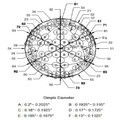•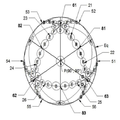•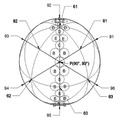••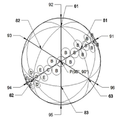•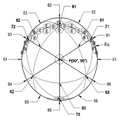•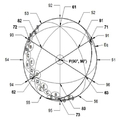•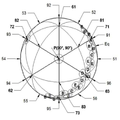•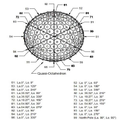•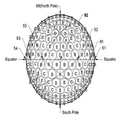••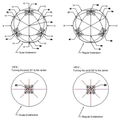## Classifications

• AHUMAN NECESSITIES
• A63SPORTS; GAMES; AMUSEMENTS
• A63BAPPARATUS FOR PHYSICAL TRAINING, GYMNASTICS, SWIMMING, CLIMBING, OR FENCING; BALL GAMES; TRAINING EQUIPMENT
• A63B37/00Solid balls; Rigid hollow balls; Marbles
• A63B37/0003Golf balls
• A63B37/0004Surface depressions or protrusions
• A63B37/0006Arrangement or layout of dimples
• AHUMAN NECESSITIES
• A63SPORTS; GAMES; AMUSEMENTS
• A63BAPPARATUS FOR PHYSICAL TRAINING, GYMNASTICS, SWIMMING, CLIMBING, OR FENCING; BALL GAMES; TRAINING EQUIPMENT
• A63B1/00Horizontal bars
• AHUMAN NECESSITIES
• A63SPORTS; GAMES; AMUSEMENTS
• A63BAPPARATUS FOR PHYSICAL TRAINING, GYMNASTICS, SWIMMING, CLIMBING, OR FENCING; BALL GAMES; TRAINING EQUIPMENT
• A63B37/00Solid balls; Rigid hollow balls; Marbles
• A63B37/0003Golf balls
• A63B37/0004Surface depressions or protrusions
• A63B37/0018Specified number of dimples
• AHUMAN NECESSITIES
• A63SPORTS; GAMES; AMUSEMENTS
• A63BAPPARATUS FOR PHYSICAL TRAINING, GYMNASTICS, SWIMMING, CLIMBING, OR FENCING; BALL GAMES; TRAINING EQUIPMENT
• A63B37/00Solid balls; Rigid hollow balls; Marbles
• A63B37/0003Golf balls
• A63B37/0004Surface depressions or protrusions
• A63B37/002Specified dimple diameter
• AHUMAN NECESSITIES
• A63SPORTS; GAMES; AMUSEMENTS
• A63BAPPARATUS FOR PHYSICAL TRAINING, GYMNASTICS, SWIMMING, CLIMBING, OR FENCING; BALL GAMES; TRAINING EQUIPMENT
• A63B2102/00Application of clubs, bats, rackets or the like to the sporting activity ; particular sports involving the use of balls and clubs, bats, rackets, or the like
• A63B2102/32Golf

## Abstract

The present invention relates to a method of dividing a golf ball surface for the arrangement of dimples having symmetry and a method of arranging dimples according to the size formed along the dividing line.
Effectively arranging the large diameter dimples in the modified spherical triangle according to the method of the present invention has the effect that the large diameter dimples have perfect symmetry across the sphere surface, thereby improving flight stability and flying distance of the golf ball.
Therefore, the present invention solves the problem of flight stability due to the lack of symmetry caused by the conventional dimple arrangement method of dividing the golf ball into spherical octahedron and arranging the large diameter dimples to improve the area ratio of the dimples. The present invention relates to a dimple arrangement method of golf balls with improved flying distance.

## Description

Golf Ball with Symmetric Dimple arrangement of Spherical Qusai-octahedron structure}

The present invention relates to a method of dividing a golf ball for symmetrically arranging dimples on the surface of a golf ball and a method of arranging dimples centering on a dividing line segment.

Dimples on the surface of the golf ball are important for aerodynamics because they play a role in increasing lift distance. Since arranging the dimples on the surface of the sphere must be arranged symmetrically with each other, the dimples are generally arranged by spherical polyhedrons having a plurality of spherical polygons by dividing the surface of the sphere using a circle. The spherical polyhedron commonly used to divide the surface of a sphere is a spherical tetrahedron consisting of four spherical equilateral triangles, a spherical tetrahedron consisting of six spherical squares, a spherical octahedron consisting of eight spherical equilateral triangles, and a spherical tetrahedron consisting of twelve spherical regular pentagons. , Spherical icosahedron consisting of 20 spherical equilateral triangles, spherical octahedron consisting of 6 spherical squares and 8 spherical equilateral triangles, and spherical 20-12 polyhedrons consisting of 20 spherical equilateral triangles and 12 spherical equilateral triangles. The dimples of golf balls that are used a lot these days are arranged in a symmetrical polyhedron with 300 to 400 dimples. Since the dimples are arranged in small sizes, many land parts (where no dimples are formed) Is formed. When the land portion is generated a lot, the dimples occupy a small area ratio on the surface of the sphere, which greatly affects the lift force.

Accordingly, many patents have been published for the method of dividing the sphere surface and the arrangement of the dimples for efficiently arranging the dimples on the surface of the sphere.

As an example, US Pat. No. 4,560,168 discloses dividing the dimples into spherical 20-12 polyhedrons by dividing the surface of a sphere into six members and connecting the midpoints of the sides in the spherical icosahedron to each other. There is a way to prevent dimples from spreading on dividing lines. Further, US 5,562,552 discloses a method of forming 60 spherical triangles by connecting the vertices of each spherical equilateral triangle at the center of each spherical equilateral triangle of spherical icosahedron, and arranging the same dimples thereon. U.S. Patent No. 5,575,477 discloses a dimple sharing area by setting a predetermined interval on each dividing line forming a spherical 20-12 polyhedron, and by arranging dimples over the existing dividing line in the area to increase the dimple area ratio to improve the flying distance. The results are posted. In addition, U. S. Patent No. 5,564, 708 discloses that the surface of a sphere is divided into spherical octahedrons or spherical hexahedrons by members, and the same six centered around the center of each spherical equilateral triangle constituting the spherical hexahedron. A method of arranging dimples and specifying the position of each dimple in a dimple arrangement of six spherical equilateral triangles adjacent to the equator is disclosed. U.S. Pat.No. 5,709,618 discloses a spherical polygon made by dividing the surface of a sphere into spherical octahedrons by making the center of one spherical equilateral triangle constituting the spherical octahedron as the pole and rotating a spherical octahedron of the same composition by 60 degrees about the pole. How to arrange the dimples in the field is posted. In addition, US Pat. No. 5,735,756 also makes a circular region inscribed with each spherical equilateral triangle formed by dividing the surface of a sphere into spherical octahedrons, arranging dimples within the region so as not to involve the dimples of other regions, and other than the above region. The dimple arrangement method has been published, which is characterized by filling dimples in all areas except the equator. US Pat. No. 6,908,403 discloses a method of dividing a surface of a sphere, characterized by dividing the surface of the sphere into members and subdividing the existing spherical octahedron into other spherical polyhedra and arranging dimples on the granular spherical polygon.

The patents describe methods of arranging dimples in a spherical polygon that forms a spherical polyhedron formed by dividing the surface of a sphere into a circle, and all spherical polyhedrons formed by the members are superimposed on the surface of a sphere having the same diameter. do. However, although the polyhedrons seem to be similar to each other, the size of each polygon is different and accordingly, the method of arranging dimples is different. Accordingly, the dimple area ratio of the dimples occupies the surface of the sphere is also different, and since the arrangement method is also different, flight characteristics such as flying distance, flight trajectory, and flight stability of the manufactured golf ball are also different.

As a prior patent, a method of improving the symmetry of dimples by arranging large diameter dimples in a spherical equilateral triangle forming a spherical octahedron according to symmetry and a method of minimizing land area by filling small land dimples with a diameter of 0.09 inch or less in the land portion There is this. However, the method using the large diameter dimple has a disadvantage in that a lot of land portions are formed, and the method using the small diameter dimple has a small lift size, so that the lift improvement effect is insignificant, and it is difficult to make a mold cavity. There is a disadvantage that the deformation of the golf ball is not produced uniformly the overall shape.

Therefore, if there is no problem in manufacturing the golf ball, and the dimple arrangement method is developed to use only the dimples with the lift effect, minimize the land area and maximize the area ratio of the dimples, the golf ball's flight stability and flying distance will be further improved. It is expected to be able.

The patents and references mentioned herein are incorporated herein by reference to the same extent as if each document was individually and clearly specified by reference.

United States Patent US 4,560,168 United States Patent US 5,562,552 United States Patent US 5,575,477 United States Patent US 5,564,708 United States Patent US 5,709,618 United States Patent US 5,735,756 United States Patent US 6,908,403

In a golf ball arranged in a spherical pseudo-octahedral composition, the dimple and land portions are arranged in perfect symmetry, and the area of the land portions is minimized to provide a golf ball with improved flying distance and flight stability.

Other objects and technical features of the present invention are more specifically shown by the following detailed description, claims and drawings.

The present invention divides the surface of the sphere with known members and reduces the land area formed when arranging the dimples on the spherical octahedron obtained through the spherical octahedron, and improves the ratio of the dimple area of the surface of the sphere. The points of contact are the same, but the dimples are arranged in a spherical pseudo-octahedral structure by dividing the sphere surface by changing the position of the vertex of the modified spherical triangle about the pole and the position of the midpoint of the spherical triangle side.

The spherical pseudooctahedron of the present invention is made of eight modified spherical triangles similar to the conventional spherical octahedron.

The modified spherical triangle constituting the spherical pseudooctahedron of the present invention differs in size from the spherical equilateral triangle constituting the conventional spherical octahedron.

In particular, the position of the dividing lines of the present invention with respect to the equator is the same as the position of the contact point with respect to the equator of the conventional members, but there is no dimple at the bisector of the sides of the spherical triangle modified to be symmetrical to the diameter of the large diameter dimples. For example, the two dimples face each other with their midpoints interposed therebetween, and the two dimples are arranged in a bisected form by each side of the deformed spherical triangle, thereby improving the symmetry of the dimples.

In addition, the present invention allows the land portion to be exactly divided into four at the vertices of four modified spherical triangles. The precisely divided land part is different from the conventional spherical octahedron in which the land part formed at the vertices of four spherical equilateral triangles is biased in one direction without being precisely divided into four.

In addition, the dimple arrangement of the present invention is a pair of bisectors of each side of the deformed spherical triangle constituting a spherical pseudooctahedron and a vertex facing each other, and a pair of pairs around the dimple having the largest diameter at the center of the deformed spherical triangle. A dimple is present adjacent to the line segment, then a dimple that is bisected to the line segment, and then a pair of dimples are adjacent to the line segment, and then again bisected to the segment. Since the dimple arrangement method is repeated in order, a spherical pseudo-octahedral dimple arrangement is minimized by minimizing land parts and having excellent symmetry of large diameter dimples.

The present invention relates to a method of dividing a golf ball for symmetrically arranging dimples on a surface of a golf ball and a method of arranging dimples around a dividing line.

Conventional golf balls have dimples arranged in spherical octahedrons formed by dividing the surface of a sphere into circumferences, so that many land portions are formed and large diameter dimples are difficult to have accurate symmetry by members.

On the contrary, the golf ball dividing method and the dimple arrangement method centering on the dividing line according to the present invention divide the golf ball into spherical pseudooctahedrons and arrange the dimples, so that dimples having a large diameter have perfect symmetry toward the equator. In addition to the overall reduction of the land portion has the effect of having a symmetry, there is an advantage that can produce a golf ball with improved flying distance and flight stability.

In addition, the golf ball dividing method and the dimple arrangement method centering on the dividing line according to the present invention, the dimple and land portion is symmetrical with only dimples of different diameters within six types, so that the dimple arrangement can be realized with a minimized land portion. The manufacturing cost of the mold cavity for the production of also has the advantage that can be saved.

Figure 1 shows the number indicating the important position of the dividing line to make the spherical pseudooctahedron of the present invention and shows the arrangement according to the dimple size of the important segment of the dividing composition.
FIG. 2 is a diagram in which symmetrical dimples are bisected on the dividing line at the midpoint (connection point) of each side of the modified spherical triangle having sides made from the start point of the dividing line segment and the connecting point of the two dividing line segments. It shows the existence of one after another. In addition, dimple A, which has the largest diameter dimple, exists in the center of the deformed spherical triangle, and the dimple-free land portion located at the vertex of the spherical triangle shows balanced symmetry by the dividing line segment.
FIG. 3 shows an arrangement of dimples of a line segment connecting each vertex of the modified spherical triangle constituting the spherical pseudooctahedron of the present invention and the midpoint of the side facing the vertex. At the center of the deformed spherical triangle is a dimple A having the largest diameter, and then two dimples of the same size are arranged facing each other with the line segment interposed therebetween, and the dimples are bisected on the segment again. Again, two dimples of the same size are arranged so that an array of dimples facing each other continues with the line segment in between. FIG. 3 illustrates an arrangement of dimples for a line segment continuously connecting one vertex and a midpoint of a side facing each other among the dimple arrangements. In particular, it is confirmed that the fourth size dimples (dimples D) at the vertices of the deformed spherical triangle have precise symmetry with each other inside the vertices, and the land portions without dimples are exactly quartered.
FIG. 4 is a diagram as shown in FIG. 3 and shows dimples arranged on a line segment connecting midpoints (connection points) of sides of a deformed spherical triangle facing a vertex in another direction.
FIG. 5 is a diagram as shown in FIG. 3 and shows dimples arranged on a line segment connecting midpoints (connection points) of sides of a deformed spherical triangle facing a vertex in another direction.
FIG. 6 is a diagram of the type shown in FIG. 3 and shows dimples arranged on a line segment connecting midpoints (connection points) of sides of a deformed spherical triangle facing a vertex in another direction. With the largest diameter dimple A in the center of each globular triangle, the midpoints of each two sides abut the equator, sharing vertices at one point location, and at two deformed globular triangles divided by the equator Shows the arrangement of the dimples by size.
FIG. 7 illustrates an arrangement of dimples on a line segment connecting midpoints (connection points) of sides of the modified spherical triangle facing vertices at different positions from FIG. 6. The dimple arrangement on the dividing line passing through the shared vertices at one point position also shows that the dimples are arranged in size according to the concept of FIG. 6.
FIG. 8 illustrates an arrangement of dimples on a line segment connecting the midpoints (connection points) of the sides of the deformed spherical triangle facing the vertex at another position, and on the dividing line passing through the shared vertices at one point position. Dimple arrangement shows that the dimples are arranged according to the size according to the concept of FIG. 6.
FIG. 9 shows a dimple arrangement according to the position and size where the other dividing line passes when the surface of the sphere is divided into spherical pseudooctahedron by making a dividing line segment different from the dividing line segment of the sphere by the members of the conventional spherical octahedron. The position of symmetry on the dividing line for is shown along with the overall sized dimple arrangement in the northern hemisphere above the equator.
FIG. 10 is a sectional view of the dimple arrangement according to the size of the spherical pseudooctahedron of FIG. 9 as seen from the equator, and shows that the new dividing line passes through the equator.
FIG. 11 shows a comparison of the dimple arrangement of the spherical pseudooctahedral segmentation composition of the present invention with the dimple arrangement of the conventional spherical octahedral divisional composition. When the size-specific dimple arrangement of the spherical pseudooctahedron is implemented at the same position in the conventional spherical octahedral dividing scheme, the dimples are not exactly divided into regular circular dividing lines, and in particular, unevenness (asymmetry) in the case of land regions without dimples. To be arranged.
FIG. 12 shows that the spherical pseudooctahedron of the present invention rotates the shared vertices of the deformed spherical triangles made by the dividing line and rotates the vertex positions so that the dimples around the vertices have an exact symmetry. The surrounding land area also shows a precise symmetry. On the contrary, the conventional spherical octahedron has a common vertex of spherical equilateral triangles created by the dividing line and the dimples around the vertex are not symmetrical and the land part is not symmetrical even if the vertex is rotated to be located at the center of the sphere. It is confirmed. All the arranged dimples have the same diameter on both sides and are arranged at the same position on the surface of the sphere of the same size.

The present invention provides a golf ball in which dimples and land portions are symmetrically arranged by dividing the surface of the golf ball into spherical pseudooctahedrons.

The spherical pseudooctahedron starts at point 51 (latitude 0 degrees, 0 degrees longitude) of the equator and points 63 (latitude 36.52 degrees, longitude 330 degrees) when any one point of the golf ball is a north pole. Gina starting at point 54 (0 latitude, 180 longitude) at the line segment and equator extending past point 83 (54.92 degrees latitude, 270 degrees longitude) and past point 62 (36.52 degrees latitude, 210 degrees longitude) and past point 83 (54.92 latitude) The first segment connected at point 83, the line segment extending to degrees 270 degrees longitude, starting at point 52 (0 latitude, 60 degrees longitude) of the equator and passing through point 61 (36.52 degrees latitude, 90 degrees longitude) Starting at point 55 (0 degrees latitude, 240 degrees longitude) of the line segment and equatorial line up to (54.92 degrees latitude, 150 degrees longitude) and past point 62 (36.52 degrees latitude, 210 degrees longitude) to point 82 (54.92 degrees latitude, longitude) 150 degrees), starting at the second line segment at point 82, point 53 (0 degrees latitude, 120 degrees longitude) at the equator, and then moving to point 61 (36.52 degrees latitude, 90 degrees longitude). B starting at point 56 (0 latitude, 300 longitude) at the line segment and equator extending to point 81 (54.92 degrees latitude, 30 degrees longitude) and past point 63 (36.52 degrees latitude, 330 degrees longitude) and past point 81 (54.92 latitudes) A modified spherical triangle comprising the north pole at the center and sharing a side with the modified spherical triangle, the other two being connected to the third line segment connected at the point 81 at the point 81. The northern hemisphere consists of three modified spherical triangles bisected by the equator and four modified spherical triangles made identically to the northern hemisphere.

Golf ball of the present invention is a dimple having a different diameter is arranged and the dimple having a different diameter is a dimple A of 0.2 to 0.2025 inches in diameter; Dimple B with a diameter of 0.1925 to 0.195 inches; Dimple C with a diameter of 0.18 to 0.1825 inches; Dimple D with a diameter of 0.17 to 0.1725 inches; Dimple E with a diameter of 0.165 to 0.1675 inches; And dimples F having a diameter of 0.13 to 0.1325 inches.

The dimple arrangement of the present invention is a method of arranging dimples on a line segment connecting one vertex of the modified spherical triangle and the bisector of the side facing the vertex, a method of arranging dimples on the sides of the modified spherical triangle, and the modified This can be explained by arranging dimples in the interior not in contact with any sides of the spherical triangle.

First, the dimple arrangement method of the sides of the deformed spherical triangle is a dimple arrangement in which one dimple is bisected by the line segments, the dimples are in contact with the bisected dimples, and two dimples are in contact with each other through the segment. It is characterized by appearing.

A single dimple D which is bisected by a line segment which is located in an inner corner of the deformed spherical triangle and connects one vertex of the deformed spherical triangle and a bisector of a side facing the vertex and contacts the vertex; Two dimples E in contact with the one dimple D and in contact with the line segment; One dimple C in contact with the two dimples E and bisected symmetrically by the line segment; Two dimples B in contact with the one dimple C and in contact with the line segment; One dimple A in contact with the two dimples B and positioned at the center of the deformed spherical triangle and bisected symmetrically by the components; Two other dimples B in contact with the one dimple A and in contact with the line segment; Another dimple B in contact with the other two dimples B and symmetrically bisected by the line segment; And dimples arranged in the order of two other dimples B which are in contact with the another dimple B and which intersect the line segment and the side facing the vertex, and are bisected symmetrically by the side facing the vertex. do.

Next, the dimples arranged on the sides of the deformed spherical triangle are characterized in that the dimples bisected by the sides are continuously arranged.

In detail, two dimples surrounding one vertex of the modified spherical triangle and abutting each other with the sides of the modified spherical triangle interposed therebetween; One dimple F in contact with the two dimples D and symmetrically bisected by the sides; One dimple E in contact with the one dimple F and bisected symmetrically by the side; One dimple B in contact with the one dimple E and in contact with the bisector of the side and symmetrically bisected by the side; Another dimple B in contact with the one dimple B and in contact with the bisector of the side and symmetrically bisected by the side; Another dimple E that is symmetrically bisected by the sides in contact with the other dimple B; Another dimple F in contact with the other dimple E and bisected symmetrically by the side; And dimples arranged in the order of two other dimples D, which are in contact with the other dimple F and surround any other vertex, and which are in contact with each other with the sides interposed therebetween.

Lastly, the dimple is arranged in a shape not in contact with any side of the deformed spherical triangle, and a dimple C is used as one dimple is arranged.

In addition, a dimple having the largest diameter is disposed in the middle of the modified spherical triangle, and two dimples having the second largest diameter are arranged to face each other with the bisector of the side of the deformed spherical triangle.

By arranging the dimples according to the dimple arrangement method of the present invention described above, 290 to 320 large diameter dimples may be arranged, and not only the dimple but also the land portion where the dimple is not located has perfect symmetry.

In particular, the land portion of the region where the vertices of the deformed spherical triangle are gathered is surrounded by the dimple D, and the connection line segment of the sides of the deformed spherical triangle and the sides of the deformed spherical triangle facing the vertex. It is divided symmetrically by the connecting line segments of the bisecting lines.

Hereinafter, a method of dividing a spherical pseudooctahedron and a symmetrical arrangement method of dimples according to the present invention will be described in detail with reference to the accompanying drawings.

In general, the mold cavity (cavity) to make a cover (cover) with the dimples of the golf ball is divided into the northern hemisphere and the southern hemisphere, the equatorial region is the mold joint line. Therefore, the mold joint line is made so that the mark is not displayed so that dimples may cross each other on the dividing line (equator) made by the crew between the northern and southern hemispheres, or the mold joint line may not be marked. Dimples are arranged only inside the spherical equilateral triangle so that the northern and southern hemispheres can be clearly distinguished and the dimples do not span the other dividing lines as well. If dimples span a dividing line other than the equator, they may change the size or position of the dimples near the equator.

In this case, dimples may not be exactly symmetrical on the basis of the dividing line forming a spherical equilateral triangle, or land portions without dimples may be formed, thereby deteriorating the flying distance and flight stability of the golf ball.

In general, the above problems are inevitably encountered in the method of arranging dimples having a large diameter and similar size in the spherical equilateral triangle constituting the spherical octahedron by dividing the surface of the sphere with the spherical octahedron. .

In order to eliminate the drawbacks and symmetrically arrange the large diameter dimples, it is necessary to adjust and change the position of the dividing line from the existing position. Of course, the triangle formed by changing the position of the dividing line becomes a modified triangle (deformed spherical triangle) different from the existing spherical equilateral triangle.

According to FIG. 9, when the modified spherical triangle has an arctic point at an arbitrary point 99 (90 degrees latitude, 90 degrees longitude) of the northern hemisphere,

Line segment and equator starting at point 51 (latitude 0 degrees, longitude 0 degrees) at the equator and passing point 63 (36.52 degrees latitude, 330 degrees longitude) to point 83 (54.92 degrees latitude, 270 degrees longitude) 54 A line segment starting at (0 degrees latitude, 180 degrees longitude) and passing through point 62 (36.52 degrees latitude, 210 degrees longitude) to point 83 (54.92 degrees latitude, 270 degrees longitude) at this point 83 to create a connecting segment , One segment of the equator starting at point 52 (latitude 0 degrees, 60 degrees longitude) at the equator and passing through point 61 (36.52 degrees latitude, 90 degrees longitude) to point 82 (54.92 degrees latitude, 150 degrees longitude) Connect another segment at point 82, starting at 55 (0 degrees latitude, 240 degrees longitude), passing point 62 (36.52 degrees latitude, 210 degrees longitude) to point 82 (54.92 degrees latitude, 150 degrees longitude) at point 82. And start at point 53 (latitude 0 degrees, longitude 120 degrees) at the equator and pass point 61 (36.52 degrees latitude, 90 degrees longitude) to point 81 (54.92 degrees latitude, longitude) Starting at point 56 (0 degrees latitude, 300 degrees longitude) of the line segment and the equator, and extending through point 63 (36.52 degrees latitude, 330 degrees longitude) to point 81 (54.92 degrees latitude, 30 degrees longitude). A segment is connected at point 81 to form another connecting segment, making the three connecting segments each side of a triangle, and sharing one side with one modified sphere triangle and the modified sphere triangle comprising a pole at the center of the triangle. And three deformed globular triangles (divided remainder in the southern hemisphere), which are bisected by the equator, are located in the northern hemisphere and are formed in the southern hemisphere equal to the four deformed globular triangles formed in the northern hemisphere. A spherical pseudooctahedron consisting of four globular triangles (total of eight deformed globular triangles) is created.

In order to achieve the dimple arrangement method according to the present invention on the spherical pseudooctahedron, the modified spherical triangle needs to be further subdivided.

According to FIG. 9, first, a line segment penetrating the vertex of the modified spherical triangle formed at the center of the sphere and the midpoint of the side facing the vertex (which is the same point as the connection point of the two line segments described above) is made.

Start at a point on the equator at 91 degrees (0 degrees latitude, 30 degrees longitude) and cross the bisecting point 81 (54.92 degrees latitude, 30 degrees longitude; the junction of the two segments) of the line segments that form the sides of the centered deformed triangle. The sphere is passed through the point 99 (90 degrees latitude, 90 degrees longitude), the pole point, and the point 94 (0 degrees latitude, 210 degrees longitude) that contacts the equator with a straight line through the point 62 (36.52 degrees latitude, 210 degrees longitude). Create a dividing line, starting at point 95 at the equator (0 degrees latitude, 270 degrees longitude) and forming a segment of the centered deformed triangular triangle at 83 (latitude 54.92 degrees, longitude 270 degrees; connection point of the two segments). ) And pass through point 99 (90 degrees latitude, 90 degrees longitude) of the pole of the sphere, passing through point 61 (36.52 degrees latitude, 90 degrees longitude), the vertex, to a point of direct contact with the equator in a straight line (0 degrees latitude, 90 degrees longitude). Create a line segment that divides the sphere, and starts at a point 93 at the equator (0 latitude, 150 degrees longitude) A vertex that passes through the bisecting point 82 (54.92 degrees latitude, 150 degrees longitude; the connection point of the two segments) of the line segments that make up the sides of the deformed spherical triangle and passes through the pole 99 of the sphere (90 degrees latitude, 90 degrees longitude). Make a line segment at point 96 (zero latitude, 330 degrees longitude) that intersects the equator in a straight line through 63 (36.52 degrees latitude, 330 degrees longitude) and subdivides the sphere.

In the three deformed quadrangular triangles facing the equator, it is made by the same method as above, but since the two segments that make up the equator side of each deformed triangular triangle are divided between the southern and northern hemispheres, the bisected two sides of the equator The point will be on the equator.

Point 61 (latitude 36.52 degrees, latitude 36.52 degrees), starting at point 51 (latitude 0 degrees, 0 degrees longitude) at the equator and passing through point 71 (19.27 degrees latitude, 30 degrees longitude), the center of the deformed spherical triangle spanning the equator Create a line segment extending up to 90 degrees longitude, starting at point 54 (0 degrees latitude, 180 degrees longitude) of the equator and ending at point 72 (19.27 degrees latitude, 150 degrees longitude) at the center of the other spherical deformation triangle over the equator. Then, a line segment is connected to a point 61 (36.52 degrees latitude and 90 degrees longitude) which is a vertex, and a connecting line segment is formed by connecting the two segments at point 61 which is a vertex.

Start at point 52 (latitude 0 degrees, 60 degrees longitude) at the equator and then point 71 (latitude 36.52 degrees, latitude 36.52 degrees longitude) through point 71 (center 19.27 degrees latitude, 30 degrees longitude) of the deformed spherical triangle over the equator. 330 degrees), and start at point 55 (zero latitude, 240 degrees longitude) of the equator, then point 73 (the latitude 19.27 degrees and 270 degrees longitude) that is the center of the other spherical transformation triangle across the equator. Then, a line segment is made up to point 63 (36.52 degrees latitude and 330 degrees longitude) which is a vertex, and another connecting line segment is formed by connecting the two segments at point 63 which is a vertex.

Point 62 (latitude 36.52 degrees), starting at point 53 (latitude 0 degrees, longitude 120 degrees) at the equator, and passing through point 72 (center 19.27 degrees latitude, 150 degrees longitude) at the center of the other spherical transformation triangle over the equator. , Create a line that extends to 210 degrees longitude, and start at point 56 (0 degrees latitude, 300 degrees longitude) of the equator, then point 73 (latitude 19.27 degrees, longitude 270) to the center of the other spherical deformation triangle across the equator. A connecting line segment connected to the vertex point 62 (latitude 36.52 degrees, the longitude 210 degrees) through the above), and another connecting line segment is formed by connecting the two segments at the vertex 62.

The three connection lines made as described above are line segments connecting straight bisectors of the sides facing through the center of the deformation sphere at the vertex of the deformation sphere triangle on the equator. It becomes a dividing line.

2 shows a method of arranging dimples in a modified spherical triangle constituting the spherical pseudooctahedron made as described above.

According to FIG. 2, first, dimple A (diameter of 0.2 inch to 0.2025 inch) is the largest diameter dimple in the center of the deformed spherical triangle constituting the spherical pseudooctahedron (points 99, 71, 72, and 73 of FIG. 9). Is placed.

The second diameter is between the bisected points (connection points of the two segments) of the opposite sides of the deformed spherical triangle centered on the pole (points 99, 71, 72 and 73 of FIG. 9). A large dimple, dimple B (0.1925 inch to 0.195 inch in diameter), is centered on the dividing line forming the sides and is bisected, with the bisectors of the sides (dots 81, 82, and 83 in FIG. 2) interposed therebetween. It is arranged to face each other.

In the deformed spherical triangle on the equatorial side, the second largest diameter dimple B (0.1925 inches to 0.195 inches in diameter) is the bisectors of the sides at the equator (points 51, 52, 53) 54, points 55 and 56) between and are bisected on the dividing line in the northern and southern hemispheres.

The dimple E (0.165 inch to 0.1675 inch in diameter), having a fifth diameter, is directed bilaterally over the dividing line and is bisected on the side (points 81, points 82, and Point 83) is placed in between, facing each displacement.

Subsequently, the smallest diameter dimple F (0.13 inches to 0.1325 inches in diameter) is disposed in the same manner.

In the same manner as above, the same dimple configuration is also bisected and divided in the dividing line even for the deformed spherical triangle in contact with the equatorial point of the side.

Fig. 3 shows the dimple arrangement on the connection line from point 92 (zero latitude, longitude 90 degrees) to point 95 (zero latitude, longitude 270 degrees) connecting the bisectors of the opposite sides of the deformed spherical triangle vertices. .

According to FIG. 3, the position and size of the dimple A is located at the center of the modified spherical triangle, as described above. The second largest diameter dimple B from the center to the side is placed next to the dimple A side by side with a connecting line connecting the bisectors of the opposite sides of the vertex, and then secondly above the connecting line. The large diameter dimple B is placed in two halves, and the second largest diameter dimple B is placed side by side with the line segments connecting the two halves of the opposite sides of the vertex. The two dimples B are the same dimples as the dimples B facing each other with the bisected points (points 81, 82, and 83 of FIGS. 2 and 3) between the sides of the modified spherical triangle.

In the direction of the dimple A at the center of the deformed spherical triangle, in the direction of the vertex (point 61 of FIG. 3), two dimples B are arranged next to each other with a line segment connecting the bisectors of the opposite sides of the vertex. Dimples C (0.18 inch to 0.1825 inches in diameter) are placed bisected above the segment, with two dimples E placed next to each other with the segments placed next to each other. Next, the fourth largest diameter dimple D (0.17 inches to 0.1725 inches in diameter) is placed directly in front of the vertex.

The dimple arrangement of the line segments connecting the bisectors of the sides facing at the vertices of the deformed spherical triangle is one dimple is bisected over the line segment, and then two dimples exist side by side with the line segment interposed therebetween. Then again one dimple is bisected over the segment, and then another dimple is bisected over the segment in a repeating and continuous fashion.

Thus, looking at a northern hemisphere, if there is a dimple on one side of the equator, two dimples on the opposite equator will show a dimple array that exists side by side with the line segment in between.

4 is another example of the dimple arrangement of the line segments connecting the bisectors of the sides facing the vertex of the modified spherical triangle. FIG. 4 illustrates a point 93 (0 latitude, 330 degrees longitude) at point 93 (zero latitude, 150 degrees longitude). Shows the dimple arrangement of the line segments connected up to). The dimple arrangement shown in FIG. 4 is identical to the arrangement of the dimples described with reference to FIG. 3.

Figure 6 connects the bisectors of the sides (point 51, point 52, point 53, point 54, point 55, and point 56 present at the equator of Figure 6) facing the vertices of the modified spherical triangle divided by the equator. Shows the dimple arrangement of the line segments. The dimple arrangement method shown in FIG. 6 is the same as the dimple arrangement method of FIG. 3.

The singularity of the dimple arrangement shown in FIG. 6 is that the four dimples D near the point 61 (36.52 degrees latitude, 90 degrees longitude) are symmetrical with the same distance from the point 61, and inside each vertex of the deformed spherical triangles. Is located in. Accordingly, it is confirmed that the land portion formed by the dimples D is equally divided by the dividing lines.

FIG. 7 shows the vertices of the modified spherical triangle divided by the equator at different locations near the equator and the bisectors of the sides facing them (points 51, 52, 53, 54, 55) And dimples located on the connecting line segment connecting point 56). The dimple arrangement method shown in FIG. 7 is the same as the dimple arrangement method of FIG. 3. In FIG. 7, as in FIG. 6, four dimples D are located at the same distance from point 62 near the point 62 (36.52 degrees latitude and 210 degrees longitude), thereby achieving perfect symmetry with respect to the point 62. It is confirmed that the resulting land portion is also surrounded by four dimples D equally divided by dividing lines.

FIG. 8 shows the vertices of the modified spherical triangle divided by the equator at another location near the equator and the bisectors of the sides facing it (points 51, 52, 53, 53 and 54 at the equator of FIG. , And points 56) show the arrangement of dimples on the line segment. The dimple arrangement of FIG. 8 is also the same as the dimple arrangement of FIG. 3, and as in FIGS. 6 and 7, four dimples D near point 63 (36.52 degrees latitude, 330 degrees longitude) are symmetrically located at the same distance from point 63. It is confirmed that the land portion generated in the region is also surrounded by four dimples D and is equally divided into four by the dividing lines.

FIG. 1 shows all of the arrangement of dimples in the segments connecting the vertices of the modified spherical triangle described above and the bisectors of the sides facing the same.

Referring to FIG. 1, the specific positions arranged according to the size and size of the dimples are well shown, and the arranged dimples are well symmetrical.

The dimple arrangement method of the spherical spherical octahedron consisting of the modified spherical triangles of the present invention is summarized as follows.

First, on each side (division line) of the deformed spherical triangle, dimples are bisected on each dividing line, and dimples are arranged next to each other (see FIG. 2).

Second, the arrangement according to the size of the dimple in the respective connecting line segments connecting the vertices of the deformed spherical triangle and the bisectors of the opposite sides thereof is such that when one dimple is bisected on the connecting line segment, the next dimple is placed on the connecting line segment. Two dimples are placed side by side, again, one dimple is bisected on the connecting line, and another dimple is alternately placed in such a way as to place one dimple (see FIG. 1).

As above, dimples are arranged for the line segments, and dimples of appropriate size are filled in the remaining spaces.

9 shows the arrangement of the dimples described above. 9 shows a dimple arrangement of the golf ball of the present invention as viewed from the pole side.

Figure 10 shows the arrangement of dimples based on the equator by rotating the dimple arrangement viewed from the pole by 90 degrees. 10, an array of large dimples of a line passing through a bisecting point (connection point of two line segments) of a dividing line that forms a spherical pseudooctahedron by forming a symmetrical dividing line based on the arrangement of the dimples by symmetry. This is done.

12 shows the result of comparing the symmetry of the dimple arrangement of the conventional spherical octahedron and the spherical pseudooctahedron of the present invention. As shown in Fig. 12, one vertex of the modified spherical triangle of the pseudo-spherical octahedron of the present invention is rotated by the angular distance from the pole (point 99) to the vertex, which is a vertex of 36.52 degrees latitude and 90 degrees longitude. Turning to, it is confirmed that the four dimples D are symmetrically arranged around the point 61 at the rotational position. In addition, it is confirmed that the land portion formed at the position is also equally divided by the dividing line so that all four dimples D can be affected in the same way during flight.

In contrast, the conventional spherical octahedron rotates the point 66 (Fig. 11 latitude 35.2643902 degrees, longitude 90 degrees) which is one vertex of the spherical equilateral triangle of the spherical octahedron by the angular distance from the pole to the vertex of the spherical equilateral triangle so that it is centered in the sphere. In turn, it can be seen that the four dimples D are located asymmetrically around point 66 in the rotational position. In addition, it can be seen that the land portion without dimples is also unevenly divided by the dividing line forming a regular spherical octahedron.

Therefore, when symmetrically arranging large diameter dimples of the present invention in the spherical equilateral triangle of the conventional spherical octahedron, the dimples are not exactly bisected by the dividing line of the spherical equilateral triangle or only a part of the dimples are arranged over the dividing line so that symmetry is achieved. There is a limit to deterioration.

The arrangement of the dimples of the golf ball is directly aerodynamically involved in lift, and in addition, greatly affects flight trajectory and flight stability. The spherical pseudo-octahedron according to the present invention has the effect of improving the flight stability of the golf ball because it eliminates the problem of symmetry of the large dimples caused by the diameter of a certain size or more generated in the regular spherical octahedron.

Specific embodiments described herein are meant to represent preferred embodiments or examples of the present invention, whereby the scope of the present invention is not limited. It will be apparent to those skilled in the art that variations and other uses of the invention do not depart from the scope of the invention described in the claims.

## Claims (13)

1. A golf ball in which dimples and land portions are symmetrically arranged by dividing the surface of a golf ball into spherical pseudooctahedrons,
The spherical pseudooctahedron starts at point 51 (latitude 0 degrees, 0 degrees longitude) of the equator and points 63 (36.52 degrees latitude and 330 degrees longitude) when any one point of the golf ball is a north pole. Line segment and equator extending from point 54 (latitude 54.92 degrees, longitude 270 degrees) through point 54 (0 degrees latitude, 180 degrees longitude) and past point 62 (36.52 degrees latitude, 210 degrees longitude) to point 83 (latitude latitude) The first segment, connected at point 83, with a segment extending up to 54.92 degrees, 270 degrees longitude, begins at point 52 (0 degrees latitude, 60 degrees longitude) of the equator and passes point 61 (36.52 degrees latitude, 90 degrees longitude). Starting at point 55 (zero latitude, 240 degrees longitude) of the line segment and equatorial line extending to 82 (54.92 degrees latitude, 150 degrees longitude) and past point 62 (36.52 degrees latitude, 210 degrees longitude), then point 82 (latitude 54.92 degrees, A second line segment connected at point 82 that extends up to 150 degrees longitude, starting at point 53 (0 degrees latitude, 120 degrees longitude) of the equator and pointing to point 61 (36.52 degrees latitude, 90 degrees longitude) B starting at point 56 (0 latitude, 300 longitude) at the line segment and equator extending to point 81 (54.92 degrees latitude, 30 degrees longitude) and past point 63 (36.52 degrees latitude, 330 degrees longitude) and past point 81 (54.92 latitudes) A modified spherical triangle comprising the north pole at the center and sharing a side with the modified spherical triangle, the other two being connected to the third line segment connected at the point 81 at the point 81. Consists of a northern hemisphere consisting of three modified spherical triangles bisected by the equator and four modified spherical triangles made identically to the northern hemisphere,
An array of dimples in a line connecting each vertex of the deformed spherical triangle and the bisector of the side facing the vertex has one dimple bisected by the line segment, abuts the one bisected dimple, and the segment A golf ball in which a dimple and land portions are symmetrically arranged by dividing a surface of a golf ball into spherical pseudooctahedrons, characterized by a repetition of a dimple arrangement in which two dimples contacting each other are mediated.
2. delete
3. 2. The dimple of claim 1, wherein the dimples arranged on the sides of the deformed spherical triangle comprise dimples bisected by the sides arranged in succession. And a golf ball in which land portions are symmetrically arranged.
4. According to claim 1, wherein the center of the deformed spherical triangle is a dimple having the largest diameter is arranged in the surface of the golf ball divided into spherical pseudo-octahedron to symmetrically arrange the dimple and land portion Golf ball.
5. 2. The golf ball surface of claim 1, wherein two dimples having the second largest diameter are arranged to face each other with the bisector of the side of the deformed spherical triangle divided into spherical pseudooctahedrons. Golf ball with symmetrical arrangement of dimples and lands.
6. The golf ball is divided into spherical pseudooctahedrons, and the dimples and the land portions are symmetrically. Arranged golf ball.
7. 7. The golf ball surface according to any one of claims 1, 3, 4, 5 or 6, wherein the deformed spherical triangle is arranged with dimples having different diameters. Golf ball with symmetrical arrangement of dimples and lands divided into spherical pseudooctahedrons.
8. 8. The method of claim 7, wherein the dimples having different diameters arranged in the modified spherical triangle comprise dimples A, having a diameter of 0.2 to 0.2025 inches; Dimple B with a diameter of 0.1925 to 0.195 inches; Dimple C with a diameter of 0.18 to 0.1825 inches; Dimple D with a diameter of 0.17 to 0.1725 inches; Dimple E with a diameter of 0.165 to 0.1675 inches; And one or more dimples selected from the group consisting of dimples F having a diameter of 0.13 to 0.1325 inches, and dividing the surface of the golf ball into spherical pseudooctahedrons to symmetrically arrange dimples and land portions.
9. The method of claim 8, wherein the dimple arrangement of the line segment connecting each vertex of the modified spherical triangle and the bisector of the side facing the vertex is located in the inner angle of the modified spherical triangle, wherein any one of the modified spherical triangle One dimple D bisected by a segment connecting the vertices of and the bisectors of the sides facing the vertices; Two dimples E in contact with the one dimple D and in contact with the line segment; One dimple C in contact with the two dimples E and bisected symmetrically by the line segment; Two dimples B in contact with the one dimple C and in contact with the line segment; One dimple A in contact with the two dimples B, positioned at the center of the deformed spherical triangle, and divided into two by the line segment; Two other dimples B in contact with the one dimple A and in contact with the line segment; Another dimple B in contact with the other two dimples B and bisected by the line segment; And another dimple B which is in contact with the another dimple B and is in contact with an intersection point at which the line facing the vertex intersects and is bisected by the side facing the vertex. A golf ball in which dimples and land portions are symmetrically arranged by dividing the surface of a golf ball into spherical pseudooctahedrons.
10. 9. The method of claim 8, wherein the dimples arranged on the sides of the deformed spherical triangles surround two vertices of the deformed spherical triangles, and two dimples D contacting each other with the sides of the deformed spherical triangle interposed therebetween; One dimple F in contact with the two dimples D and bisected symmetrically by the sides; One dimple E in contact with the one dimple F and bisected symmetrically by the side; One dimple B in contact with the one dimple E, in contact with the bisector of the side, and symmetrically bisected by the side; Another dimple B in contact with the one dimple B, in contact with the bisector of the side, and bisected symmetrically by the side; Another dimple E contacting the other dimple B and bisected symmetrically by the side; Another dimple F in contact with the other dimple E and bisected symmetrically by the side; And spherical pseudooctahedrons in contact with the other dimples F, surrounding one of the other vertices, and arranged in the order of two other dimples D contacting each other with the sides interposed therebetween. Golf ball with symmetrical arrangement of dimples and lands.
11. The golf ball is divided into spherical pseudooctahedrons, and the dimples and land portions are symmetrical. Arranged golf ball.
12. The land portion of the site where the vertices of the deformed spherical triangles are collected is surrounded by dimples D, and the vertex of the sides of the deformed spherical triangles and any vertex and the vertex of the deformed spherical triangles. A golf ball in which dimples and lands are symmetrically arranged by dividing a surface of a golf ball into spherical pseudooctahedrons, characterized by being symmetrically divided by connecting line segments of bisecting sides of opposite sides.
13. The golf ball of claim 1, wherein the number of the dimples is 290 to 320, and the dimples and land portions are symmetrically arranged by dividing the surface of the golf ball into spherical pseudooctahedrons.
KR1020170113181A 2017-09-05 2017-09-05 Golf Ball with Symmetric Dimple arrangement of Spherical Qusai-octahedron structure KR102023971B1 (en)

## Priority Applications (1)

Application Number Priority Date Filing Date Title
KR1020170113181A KR102023971B1 (en) 2017-09-05 2017-09-05 Golf Ball with Symmetric Dimple arrangement of Spherical Qusai-octahedron structure

## Applications Claiming Priority (2)

Application Number Priority Date Filing Date Title
KR1020170113181A KR102023971B1 (en) 2017-09-05 2017-09-05 Golf Ball with Symmetric Dimple arrangement of Spherical Qusai-octahedron structure
US15/939,959 US20190070465A1 (en) 2017-09-05 2018-03-29 Golf ball with symmetric dimple arrangement of spherical quasi-octahedron structure

## Publications (2)

Publication Number Publication Date
KR20190026374A KR20190026374A (en) 2019-03-13
KR102023971B1 true KR102023971B1 (en) 2019-09-23

# Family

## Family Applications (1)

Application Number Title Priority Date Filing Date
KR1020170113181A KR102023971B1 (en) 2017-09-05 2017-09-05 Golf Ball with Symmetric Dimple arrangement of Spherical Qusai-octahedron structure

## Country Status (2)

US (1) US20190070465A1 (en)
KR (1) KR102023971B1 (en)

## Family Cites Families (21)

* Cited by examiner, † Cited by third party
Publication number Priority date Publication date Assignee Title
US4560168A (en) 1984-04-27 1985-12-24 Wilson Sporting Goods Co. Golf ball
US4765626A (en) * 1987-06-04 1988-08-23 Acushnet Company Golf ball
JP2569776B2 (en) * 1988-12-02 1997-01-08 ブリヂストンスポーツ株式会社 Golf ball
JP2918671B2 (en) * 1990-10-12 1999-07-12 住友ゴム工業株式会社 Golf ball
JP2844905B2 (en) * 1990-11-07 1999-01-13 住友ゴム工業株式会社 Golf ball
US5253872A (en) * 1991-12-11 1993-10-19 Ben Hogan Co. Golf ball
JP2716932B2 (en) * 1993-12-07 1998-02-18 住友ゴム工業株式会社 Golf ball
KR960016742B1 (en) 1994-01-25 1996-12-20 이세권 Golf ball
US5415410A (en) * 1994-02-07 1995-05-16 Acushnet Company Three parting line quadrilateral golf ball dimple pattern
KR970005338B1 (en) 1994-09-06 1997-04-15 이세권 Golf ball
US5562552A (en) 1994-09-06 1996-10-08 Wilson Sporting Goods Co. Geodesic icosahedral golf ball dimple pattern
US5709618A (en) 1996-04-12 1998-01-20 Ilya Co. Ltd. Golf ball
US5735756A (en) 1996-09-10 1998-04-07 Lisco, Inc. Golf ball and dimple pattern forming process
US5957786A (en) * 1997-09-03 1999-09-28 Acushnet Company Golf ball dimple pattern
US5957787A (en) * 1998-07-01 1999-09-28 Woohak Leispia Inc. Golf ball having annular dimples
JP4519978B2 (en) * 2000-03-08 2010-08-04 Ｓｒｉスポーツ株式会社 golf ball
JP4672210B2 (en) * 2001-08-21 2011-04-20 Ｓｒｉスポーツ株式会社 Golf ball
KR100506196B1 (en) 2004-02-13 2005-07-28 주식회사 볼빅 Golf ball
US20120015761A1 (en) * 2010-07-19 2012-01-19 Madson Michael R Golf ball having multiple identical staggered parting lines
KR101367277B1 (en) * 2012-04-26 2014-02-26 주식회사 볼빅 Dimple arrangement on the surface of a golf ball and the golf ball thereof

## Also Published As

Publication number Publication date
US20190070465A1 (en) 2019-03-07
KR20190026374A (en) 2019-03-13

## Similar Documents

Publication Publication Date Title
US6471605B2 (en) Golf ball with pyramidal protrusions
US5080367A (en) Golf ball
DE60030469T2 (en) Golfball with tubular grid structure
US8632424B2 (en) Golf ball with improved flight performance
US6358161B1 (en) Golf ball dimple pattern
US5145180A (en) Golf ball
EP0476223A1 (en) Golf ball
AU598401B2 (en) Golf balls
US4949976A (en) Multiple dimple golf ball
US7156757B2 (en) Golf ball with improved flight performance
JP2843557B2 (en) Golf ball
US4880241A (en) Golf ball
US5046742A (en) Golf ball
CN1190245C (en) Golf ball dimples with curvature continuity
EP0587285A1 (en) Golf ball with novel dimple pattern
US4804189A (en) Multiple dimple golf ball
US7513839B2 (en) Golf ball with improved dimple pattern
US5060954A (en) Multiple dimple golf ball
JP3726231B2 (en) golf ball
JP2004236745A (en) Golf ball
US6632150B1 (en) Golf ball having a sinusoidal surface
JP2805383B2 (en) Improved dimple pattern
US5879245A (en) Golf ball
CA1287845C (en) Golf ball
US5957787A (en) Golf ball having annular dimples

## Legal Events

Date Code Title Description
A201 Request for examination
E902 Notification of reason for refusal
E701 Decision to grant or registration of patent right
GRNT Written decision to grant